# Solving Large Systems of Linear Simultaneous Equations

2046 words (8 pages) Essay

18th Sep 2017 Mathematics Reference this

Disclaimer: This work has been submitted by a university student. This is not an example of the work produced by our Essay Writing Service. You can view samples of our professional work here.

Any opinions, findings, conclusions or recommendations expressed in this material are those of the authors and do not necessarily reflect the views of UKEssays.com.

NICOLE LESIRIMA

METHODS OF SOLVING LARGE SYSTEMS OF LINEAR SIMULTANEOUS EQUATIONS

1. PROJECT DESCRIPTION

Linear systems simulate real-world problems using applied numerical procedure. The main aim of this project is to consider what factors affect the efficiency of the various methods of solving linear simultaneous equations. So far, one of the main factors is rounding errors that can produce inaccurate solutions. Moreover, MATLAB programs have been produced to time the calculation speed to determine the efficiency of the methods. Generally, these methods are subdivided into two; direct and iterative methods. Direct methods are commonly used to solve small systems of equations. The iterative methods are used to solve real-world problems that produce systems of equations for which the coefficient matrices are sparse.

The relevance of studying these methods have its real world applications. The real world applications can be seen in various fields such as science and engineering, accounting and finance, business management and in operational research. The approach provides a logical framework for solving complex decisions in a wide range of industries. The advantage is that, decisions are founded on data analysis.

If you need assistance with writing your essay, our professional essay writing service is here to help!

Environmentalists and meteorologists may use large systems of simultaneous linear equations to predict future outcomes. For instance, to predict weather patterns or climate change, a large volume of data is collected over a long span of time on many variables including, solar radiation, carbon emissions and ocean temperatures. Queen Mary University of London (2015). This data is represented in the form of a transition matrix that has to be row reduced into a probability matrix that can then be used in the prediction of climate change.

The objective of an enterprise is to maximize returns while maintaining minimum costs. Whereas the use of large systems of simultaneous linear equations may provide a basis for evidence based business decision making in an enterprise, it is important to know which linear systems are most appropriate in order to minimize undesirable outcomes for an enterprise.

1. PROJECT REPORT OUTLINE

Chapter 1

Introduction

Large systems of linear simultaneous equations are used to simulate real-world problems using applied numerical procedure. The real world applications can be seen in various fields such as science and engineering, accounting and finance, business management. The approach provides a logical framework for solving complex decisions in a wide range of industries. The advantage is that decisions are founded on data analysis. The aim of this project is to explore the efficiency of a large systems of linear simultaneous equations in the optimal decision making of an enterprise.

Chapter 2

Direct Methods: Gaussian Elimination and LU Factorisation

Direct methods of solving linear simultaneous equations are introduced. This chapter will look at the Gaussian Elimination and LU Factorisation methods. Gaussian Elimination involves representing the simultaneous equations in an augmented form, performing elementary row operations to reduce the upper triangular form and finally back substituting to form the solution vector. LU Factorisation on the other hand is where a matrix A finds a lower triangular matrix L and an upper triangular matrix U such that A = LU. The purpose of this lower triangular matrix and upper triangular matrix is so that the forward and backward substitutions can be directly applied to these matrices to obtain a solution to the linear system. An operation count and computing times using MATLAB is calculated so as to determine the best method to use.

Chapter 3

Cholesky Factorisation

Introduction to the Cholesky method. This is a procedure whereby the matrix A is factorised into the product of a lower triangular matrix and its transpose; the forward and backward substitutions can be directly applied to these matrices to obtain a solution. A MATLAB program is written to compute timings. A conclusion can be drawn by comparing the three methods and determining which is the most suitable method that will produce the most accurate result as well as take the shortest computing time.

Chapter 4

Iterative Methods: Jacobi Method and Gauss-Seidel

This chapter will introduce the iterative methods that are used to solve linear systems with coefficient matrices that are large and sparse. Both methods involve splitting the matrix A into lower triangular, diagonal and upper triangular matrices L, D, U respectively. The main difference comes down to the way the x values are calculated. The Jacobi method uses the previous x values (n) to calculate the next iterated x values (n+1). The Gauss-Seidel uses the new x value (n+1) to calculate the x2 value.

Chapter 5

Successive Over Relaxation and Conjugate Gradient

Other iterative methods are introduced. The Successive Over Relaxation method over relaxes the solution at each iteration. This method is calculated using the weighted sum of the values from the previous iteration and the values form the Gauss-Seidel method at the current iteration. The Conjugate Gradient method involves improving the approximated value of xk to the exact solution which may be reached after a finite number of iterations usually smaller than the size of the matrix.

Chapter 6

Conclusion

All the project findings and results are summarised in this chapter. Conclusion can be made from both direct methods and iterative methods whereby the most accurate method with the shortest computing time can be found. Drawbacks from each method will be mentioned as well its suitability for solving real world problems.

1. PROGRESS TO DATE

The project to date has covered the direct methods of solving simultaneous equations.

Gaussian Elimination

This involves representing the simultaneous equations in an augmented form, performing elementary row operations to reduce the upper triangular form and finally back substituting to form the solution vector. For example, to solve an mxn matrix:

Ax = b

The aim of the Gaussian elimination is to manipulate the augmented matrix [A|b] using elementary row operations; by adding a multiple of the pivot rows to the rows beneath the pivot row i.e. Riâ€¬â€¬â€¬â€¬ïƒ  Ri +kRj. Once the augmented matrix is in the row echelon form, the solution is found using back substitution.

The following general matrix equation has been reduced to row echelon form: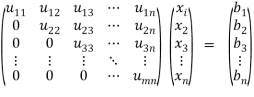This corresponds to the linear system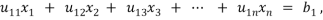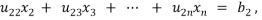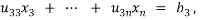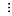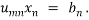Rearranging the final solution is given by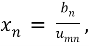For all other equations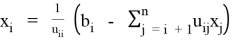i = n – 1, . . .,

The operation count and timing the Gaussian Elimination was performed. The total number of operations for an nxn matrix using the Gaussian elimination is with O(N3).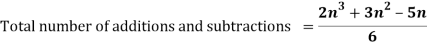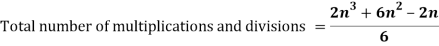LU Factorisation

This is where a matrix A finds a lower triangular matrix L and an upper triangular matrix U such that A = LU. The purpose of this lower triangular matrix and upper triangular matrix is so that the forward and backward substitutions can be directly applied to these matrices to obtain a solution to the linear system.

In general,

L and U is an m x n matrix:

L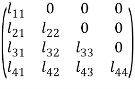U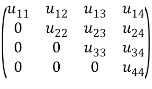For higher order matrices, we can derive the calculation of the L and U matrices. Given a set of n elementary matrices E1, E2,…, Enapplied to matrix A, row reduce in row echelon form without permuting rows such that A can be written as the product of two matrices L and U that is

A = LU,

Where

U = En…E2E1A,

L = E1-1 E2-1…En-1

For a general nxn matrix, the total number of operations is O(N3). A Matlab program has been produced to time the LU Factorisation. So far, this method has proven more efficient than the Gaussian Elimination.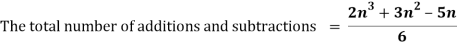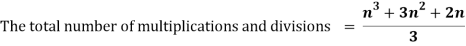Cholesky Factorisation

This is a procedure whereby the matrix A is factorised into the product of a lower triangular matrix and its transpose i.e. A = LLT  or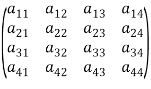=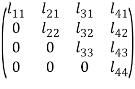The Cholesky factorisation is only possible if A is a positive definite. Forward and backward substitution is employed in finding the solutions.

The method was also timed at it can be concluded that it is the most effective and efficient direct method for solving simultaneous equations.

The indirect methods have been introduced with a short outline of what each method entails.

1. Work Still to be Completed

As from the objectives layed out from the terms of reference, the following are the objectives that are yet to be completed.

NICOLE LESIRIMA

METHODS OF SOLVING LARGE SYSTEMS OF LINEAR SIMULTANEOUS EQUATIONS

1. PROJECT DESCRIPTION

Linear systems simulate real-world problems using applied numerical procedure. The main aim of this project is to consider what factors affect the efficiency of the various methods of solving linear simultaneous equations. So far, one of the main factors is rounding errors that can produce inaccurate solutions. Moreover, MATLAB programs have been produced to time the calculation speed to determine the efficiency of the methods. Generally, these methods are subdivided into two; direct and iterative methods. Direct methods are commonly used to solve small systems of equations. The iterative methods are used to solve real-world problems that produce systems of equations for which the coefficient matrices are sparse.

The relevance of studying these methods have its real world applications. The real world applications can be seen in various fields such as science and engineering, accounting and finance, business management and in operational research. The approach provides a logical framework for solving complex decisions in a wide range of industries. The advantage is that, decisions are founded on data analysis.

Environmentalists and meteorologists may use large systems of simultaneous linear equations to predict future outcomes. For instance, to predict weather patterns or climate change, a large volume of data is collected over a long span of time on many variables including, solar radiation, carbon emissions and ocean temperatures. Queen Mary University of London (2015). This data is represented in the form of a transition matrix that has to be row reduced into a probability matrix that can then be used in the prediction of climate change.

The objective of an enterprise is to maximize returns while maintaining minimum costs. Whereas the use of large systems of simultaneous linear equations may provide a basis for evidence based business decision making in an enterprise, it is important to know which linear systems are most appropriate in order to minimize undesirable outcomes for an enterprise.

1. PROJECT REPORT OUTLINE

Chapter 1

Introduction

Large systems of linear simultaneous equations are used to simulate real-world problems using applied numerical procedure. The real world applications can be seen in various fields such as science and engineering, accounting and finance, business management. The approach provides a logical framework for solving complex decisions in a wide range of industries. The advantage is that decisions are founded on data analysis. The aim of this project is to explore the efficiency of a large systems of linear simultaneous equations in the optimal decision making of an enterprise.

Chapter 2

Direct Methods: Gaussian Elimination and LU Factorisation

Direct methods of solving linear simultaneous equations are introduced. This chapter will look at the Gaussian Elimination and LU Factorisation methods. Gaussian Elimination involves representing the simultaneous equations in an augmented form, performing elementary row operations to reduce the upper triangular form and finally back substituting to form the solution vector. LU Factorisation on the other hand is where a matrix A finds a lower triangular matrix L and an upper triangular matrix U such that A = LU. The purpose of this lower triangular matrix and upper triangular matrix is so that the forward and backward substitutions can be directly applied to these matrices to obtain a solution to the linear system. An operation count and computing times using MATLAB is calculated so as to determine the best method to use.

Chapter 3

Cholesky Factorisation

Introduction to the Cholesky method. This is a procedure whereby the matrix A is factorised into the product of a lower triangular matrix and its transpose; the forward and backward substitutions can be directly applied to these matrices to obtain a solution. A MATLAB program is written to compute timings. A conclusion can be drawn by comparing the three methods and determining which is the most suitable method that will produce the most accurate result as well as take the shortest computing time.

Chapter 4

Iterative Methods: Jacobi Method and Gauss-Seidel

This chapter will introduce the iterative methods that are used to solve linear systems with coefficient matrices that are large and sparse. Both methods involve splitting the matrix A into lower triangular, diagonal and upper triangular matrices L, D, U respectively. The main difference comes down to the way the x values are calculated. The Jacobi method uses the previous x values (n) to calculate the next iterated x values (n+1). The Gauss-Seidel uses the new x value (n+1) to calculate the x2 value.

Chapter 5

Successive Over Relaxation and Conjugate Gradient

Other iterative methods are introduced. The Successive Over Relaxation method over relaxes the solution at each iteration. This method is calculated using the weighted sum of the values from the previous iteration and the values form the Gauss-Seidel method at the current iteration. The Conjugate Gradient method involves improving the approximated value of xk to the exact solution which may be reached after a finite number of iterations usually smaller than the size of the matrix.

Chapter 6

Conclusion

All the project findings and results are summarised in this chapter. Conclusion can be made from both direct methods and iterative methods whereby the most accurate method with the shortest computing time can be found. Drawbacks from each method will be mentioned as well its suitability for solving real world problems.

1. PROGRESS TO DATE

The project to date has covered the direct methods of solving simultaneous equations.

Gaussian Elimination

This involves representing the simultaneous equations in an augmented form, performing elementary row operations to reduce the upper triangular form and finally back substituting to form the solution vector. For example, to solve an mxn matrix:

Ax = b

The aim of the Gaussian elimination is to manipulate the augmented matrix [A|b] using elementary row operations; by adding a multiple of the pivot rows to the rows beneath the pivot row i.e. Riâ€¬â€¬â€¬â€¬ïƒ  Ri +kRj. Once the augmented matrix is in the row echelon form, the solution is found using back substitution.

The following general matrix equation has been reduced to row echelon form:This corresponds to the linear systemRearranging the final solution is given byFor all other equationsi = n – 1, . . .,

The operation count and timing the Gaussian Elimination was performed. The total number of operations for an nxn matrix using the Gaussian elimination is with O(N3).LU Factorisation

This is where a matrix A finds a lower triangular matrix L and an upper triangular matrix U such that A = LU. The purpose of this lower triangular matrix and upper triangular matrix is so that the forward and backward substitutions can be directly applied to these matrices to obtain a solution to the linear system.

In general,

L and U is an m x n matrix:

LUFor higher order matrices, we can derive the calculation of the L and U matrices. Given a set of n elementary matrices E1, E2,…, Enapplied to matrix A, row reduce in row echelon form without permuting rows such that A can be written as the product of two matrices L and U that is

A = LU,

Where

U = En…E2E1A,

L = E1-1 E2-1…En-1

For a general nxn matrix, the total number of operations is O(N3). A Matlab program has been produced to time the LU Factorisation. So far, this method has proven more efficient than the Gaussian Elimination.Cholesky Factorisation

This is a procedure whereby the matrix A is factorised into the product of a lower triangular matrix and its transpose i.e. A = LLT  or=The Cholesky factorisation is only possible if A is a positive definite. Forward and backward substitution is employed in finding the solutions.

The method was also timed at it can be concluded that it is the most effective and efficient direct method for solving simultaneous equations.

The indirect methods have been introduced with a short outline of what each method entails.

1. Work Still to be Completed

As from the objectives layed out from the terms of reference, the following are the objectives that are yet to be completed.

1. Week 13 – 16: Evaluating the convergence rate of the iterative methods in detail as well as finding out which method improves the solution efficiency.  Production of MATLAB programs analysing the different methods and other methods. Over the next 3 weeks, the conditions for convergence will be analysed. One of the most important conditions that will be studied is the spectral radius. This is a condition applied on the indirect methods to determine how fast or slow a method takes to achieve the state of convergence. Moreover, the project will also produce Matlab programs for the iterative methods and employ the spectral radius on these programs to determine the speed of convergence for large sparse matrices.
1. Weeks 17 – 19: Introduction to the Successive Over-Relaxation (SOR) method and the Conjugate Gradient method. Successive Over-Relaxation method improves the rate of convergence of the Gauss-Siedel method by over-relaxing the solution at every iteration. While the Conjugate Gradient improves the approximated value of x to the exact solution. Matlab programs will be produced for the two methods together with the speed of convergence of different sizes of matrices.
1. Week 20 – 24:Writing the findings and conclusions of the report, finalising on the bibliography and doing a review of the project as a whole.  Preparing oral and poster presentation.

## Related Services

View all

### DMCA / Removal Request

If you are the original writer of this essay and no longer wish to have your work published on the UKDiss.com website then please:

Related Services

Related Lectures